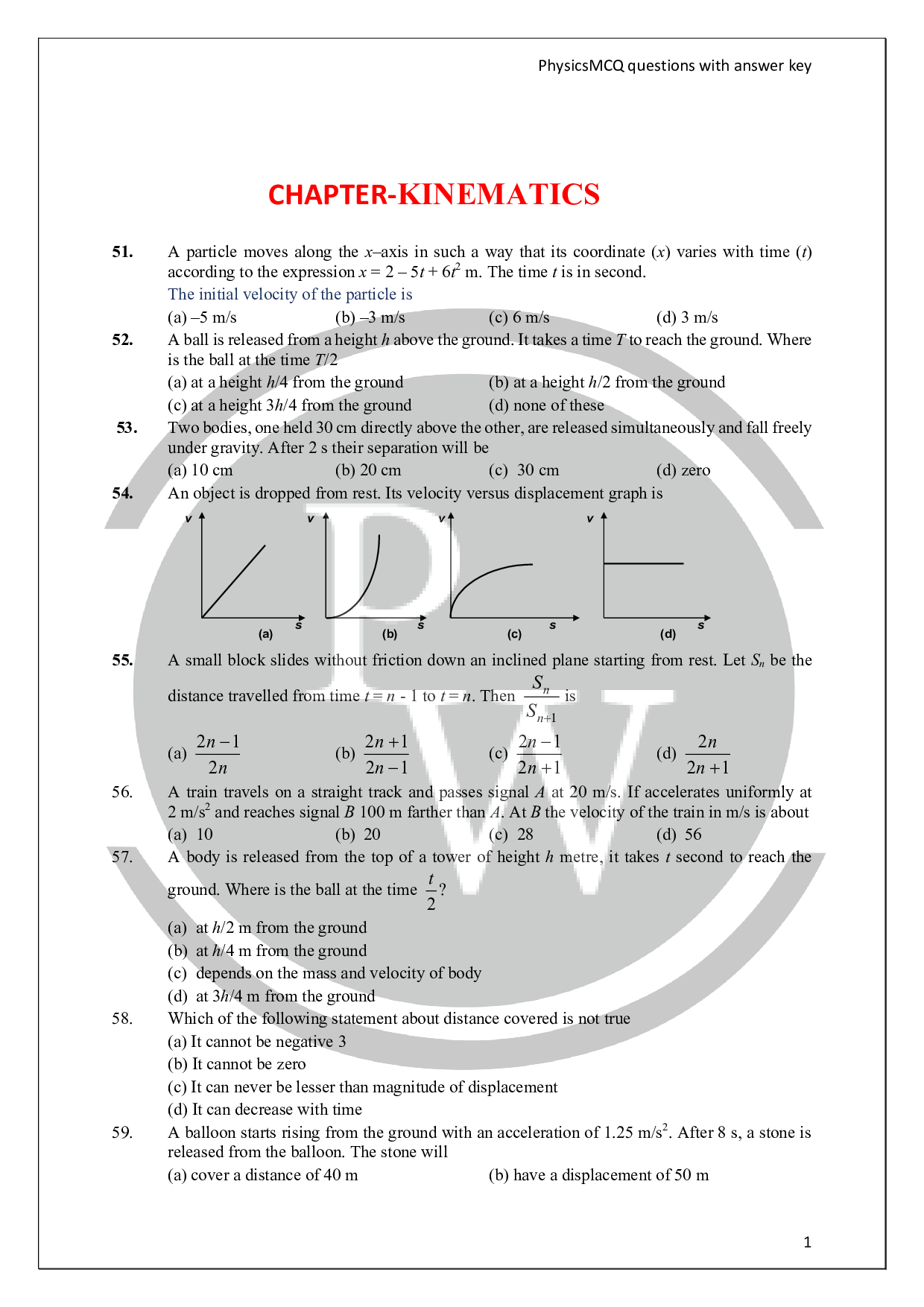# Kinematics Questions

As a first step in studying classical mechanics, This chapter describe the motion of an object while ignoring the interaction with external agents that might be causing or modifying that motion. This portion of classical mechanics is called kinematics. To facilitate the learning process for the students we have split kinematics in to two parts.

Kinematics – II deals with calculus based kinematics and non-uniform motion. Physics Wallah consists of summarized text coupled with sufficient number of solved examples of varying difficulties, which enables the students to develop problem solving ability along with emphasis on physical concept.

The end–of–chapter problems are categorized into four section, namely Exercise – I (objectives where only one of the option is correct), to help the student assess his understanding of the concept and further improvise on his problem solving skills. Every possible attempt has been made to make the sheet of questions flawless. Do check out chapter wise Physics Questions prepared by Physics Wallah. Students can also access the Class 11 Kinematics Notes from here.

If any students need to take the online test to check their concepts or undertstanding then they can visit Quiz for Kinematics.Q1. What are the five significant kinematic quantities?

Ans. The five significant kinematic quantities are displacement (x-x0), time (t), initial velocity (v0), final velocity (v), and constant acceleration (a). These quantities are commonly included when describing the position and motion of an object.

Q2. Are kinematics tough?

Ans. This is not a challenging chapter, though some of the concepts, especially differentiation, integration, and vectors. Relative motion and acceleration of a particle in uniform circular motion would feel new.

Q3. Can velocity be negative?

Ans. An object which moves in the negative direction has a negative velocity. If the object slows down, its acceleration vector is directed in the opposite direction as its motion (in this case, a positive acceleration).

Q4. What are kinematics examples?

Ans. Examples of kinematics

• The movement of a vehicle in a straight line (constant velocity motion)
• A person skydiving (Free fall)
• An apple that falls from a tree (free-fall)
• A rocket flying towards space (vertical motion)
• A plane flying (uniform accelerated motion)
• A three-shot point in basketball (projectile motion)

Q5. What are the four equations of motion?

Ans. They are often referred to as the SUVAT equations, where "SUVAT" is an acronym from the variables: s = displacement, u = initial velocity, v = final velocity, a = acceleration, and t = time.

Q6. Is kinematics important for JEE?

Ans. It is one of the essential chapters in the Physics syllabus of JEE Main and JEE Advanced. About 2-3 questions are always asked from this chapter in the exam.

#### Find below multiple choice questions pdf of Kinematics questions